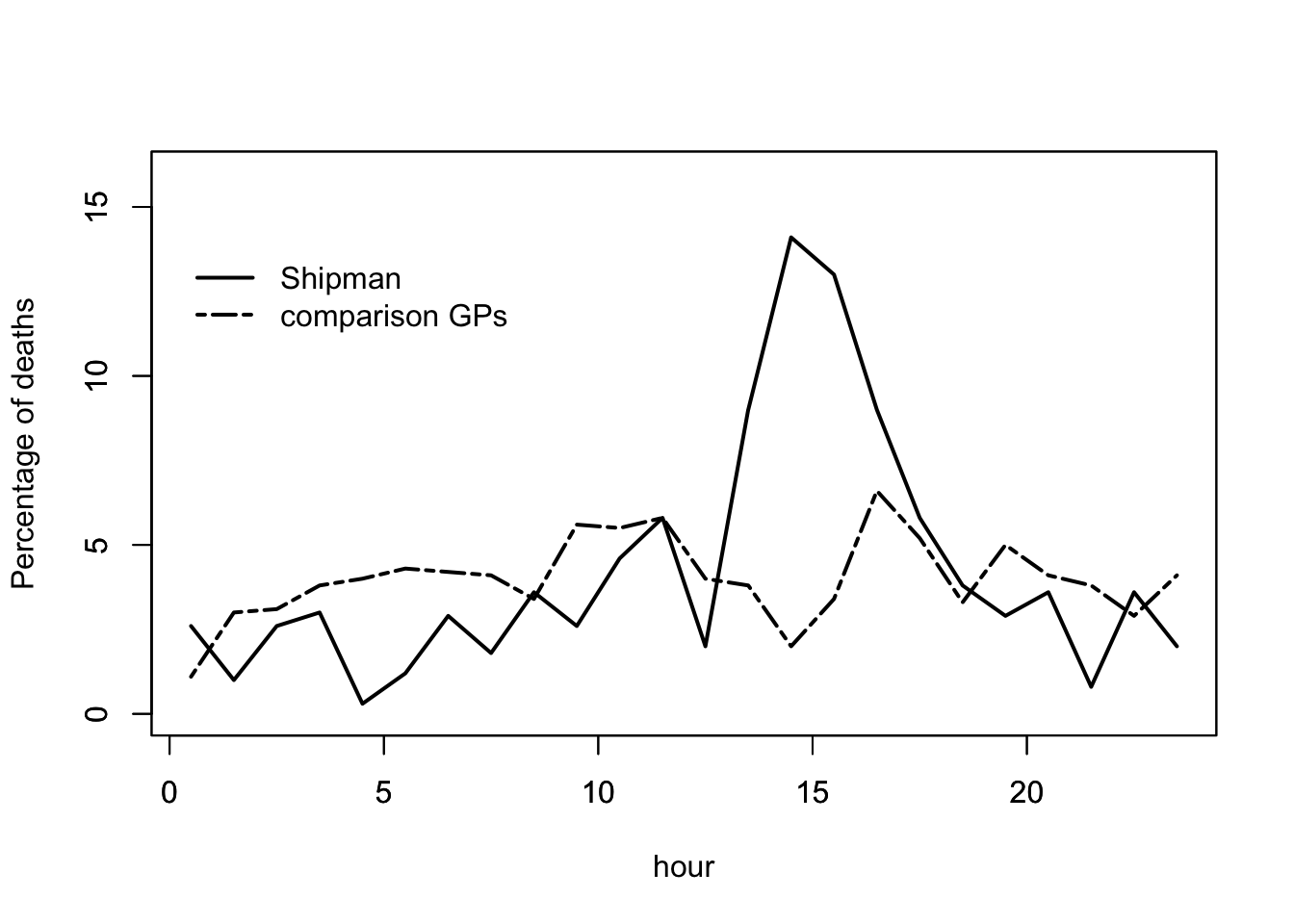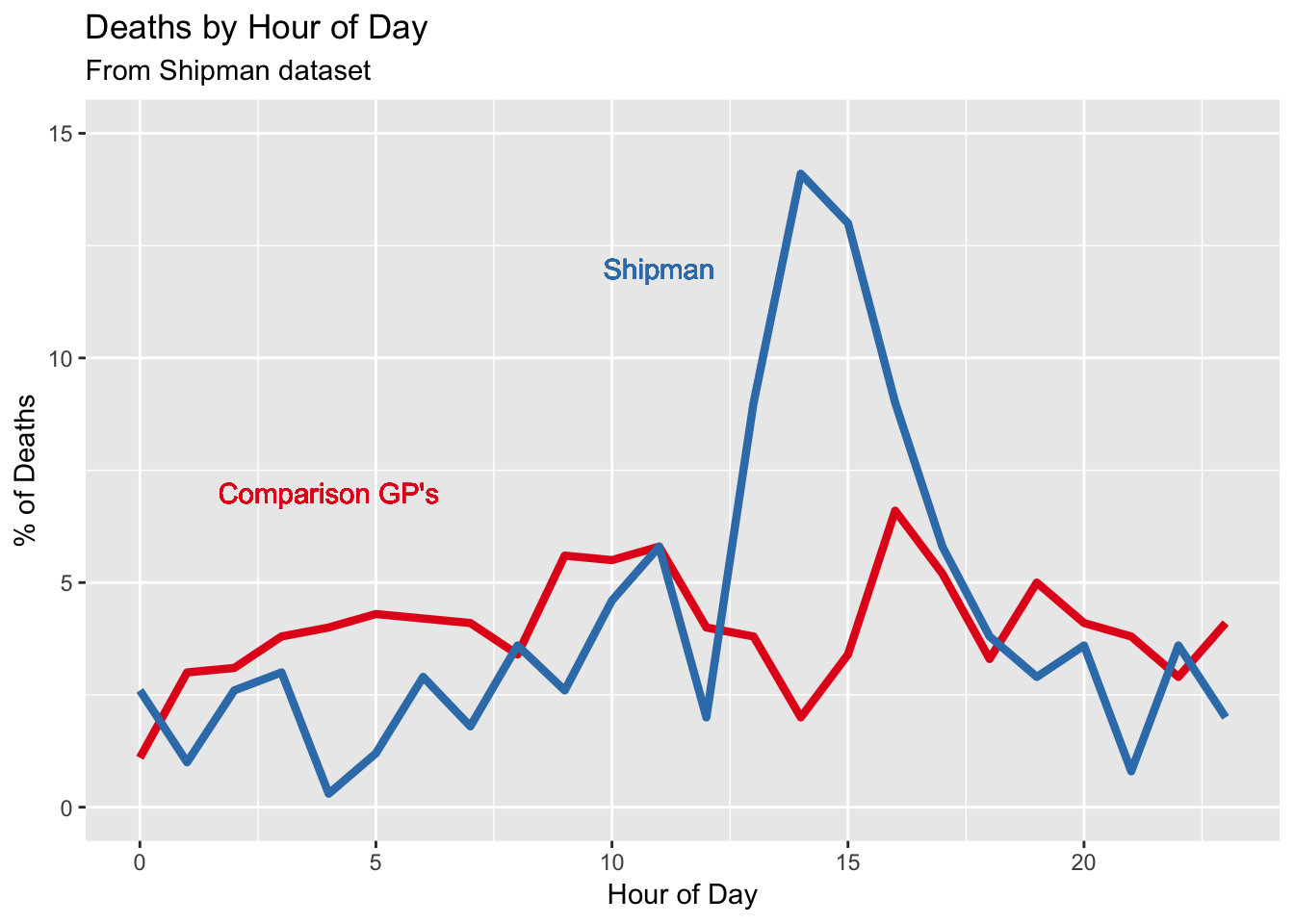At what time of day did Harold Shipman’s victims die?

Data are contained in 0-2-shipman-times-x.csv, and taken from Harold Shipman’s Clinical Practice 1974-1998: A Clinical Audit Commissioned by the Chief Medical Officer, by Richard Baker, page 40, Figure 5.2, derived from the cremation forms of Shipman’s victims.

library(ggplot2)

attach(shipmantimes)

Plot using R Base graphics

plot(Hour+0.5,Shipman,type="l",lwd=2,ylab="Percentage of deaths",xlab="hour",ylim=c(0,16))
par(new=T)
plot(Hour+0.5,Comparison,type="l",lwd=2,xlab="",ylab="",ylim=c(0,16),lty=6)
legend(0,14,c("Shipman","comparison GPs"),lty=c(1,6),bty="n",lwd=2)Now using ggplot2

p <- ggplot(shipmantimes, aes(x=Hour, y)) + ylim(0,15) # constructs initial plot object, p
p <- p + geom_line(aes(y = Comparison, col = "Comparison GPs"), size=1.5) # adds a y-series
p <- p + geom_line(aes(y = Shipman, col = "Shipman"), size=1.5) # adds a y-series
p <- p +  labs(title="Deaths by Hour of Day", subtitle="From Shipman dataset", y="% of Deaths", x="Hour of Day") # Adds title, subtitle
p <- p + scale_colour_brewer(palette = "Set1") # sets the colour palette
p <- p + theme(legend.position="none")#, legend.box = "horizontal") # removes the legend

p <- p + geom_text(aes(x = 11, y = 12, label = "Shipman", color = "Shipman"))
p <- p + geom_text(aes(x = 4, y = 7, label = "Comparison GP's", color = "Comparison GPs"))
pFigure 0.2. The time at which Harold Shipman’s patients died, compared to the times at which patients of other local general practitioners died. The pattern does not require sophisticated statistical analysis.

Shipman used to make his home visits after lunch. For a chilling descrption of how he murdered his victims, see any of the detailed case histories.